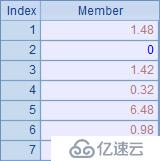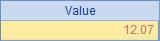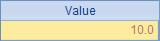# 如何处理报表中的舍位平衡

1. 单向舍位平衡

A

B

C

1

[1.48,0,1.42,0.32,6.48,0.98,1.39]

=A1.sum()

2

=A1.(round(~))

=round(B1)

=A2.sum()

A1的序列中存储了一些数据,在B1中计算了它们的合计值，结果如下：(1) 将平衡差整理到第一个数据中。即：

A

B

C

1

[1.48,0,1.42,0.32,6.48,0.98,1.39]

=A1.sum()

2

=A1.(round(~))

>A2(1)+=round(B1)-A2.sum()

=A2.sum()

B2中，把平衡差折算到舍位后的第一个数据中。整理后，在C2中重新计算了舍位平衡处理后的合计值。A2和C2中的结果如下：(2) 将平衡差按照“最小调整值”，对绝对值比较大的数据进行分担调整。

A

B

C

1

[1.48,0,1.42,0.32,6.48,0.98,1.39]

=A1.sum()

2

=A1.(round(~))

=round(B1)-A2.sum()

=sign(B2)

3

=A1.psort@z(abs(~))

>abs(B2).run(A2(A3(#))+=C2)

=A2.sum()

A3中，根据原始数据的绝对值从大到小做了一个排序，结果就是排序后的序号。

B3是最主要的，因为只是取整操作，所以B2中的偏差绝对值是多少，就调整几个数。以此循环，依照原始值的绝对值大小，依次分配最小调整值。

C3是对调整后的A2重新验证了合计值。